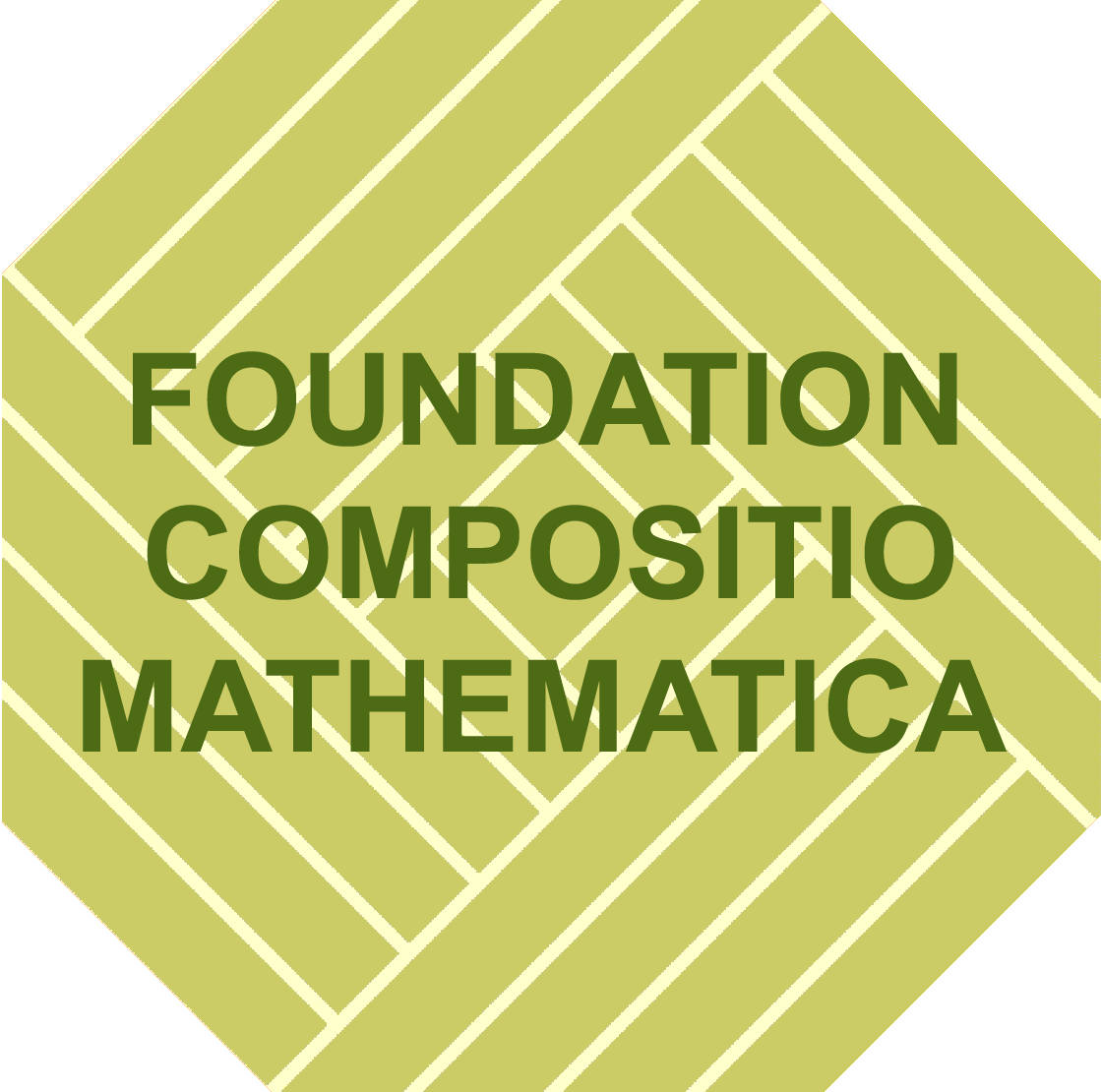## Winter Meeting in Algebra and Geometry

Rome : December 18 - 20 2023

## Speakers

Name Institution
Jennifer Balakrishnan Boston University
Andrea Bruno Roma Tre University
Ziyang Gao Leibniz University Hannover
Alex Küronya Goethe-Universität Frankfurt
Emanuele Macrì Université Paris-Saclay
Gabriele Mondello Sapienza Unviersity of Rome
Paolo Salvatore University of Rome "Tor Vergata"
Wolfgang Soergel Albert-Ludwigs-Universität Freiburg

## Venue

Aula Urbano VIII dell’Argiletum (Università Roma Tre, Dipartimento di Architettura), Via della Madonna dei Monti, 40 – 00184 Roma

History. Since 2011, the Algebra and Geometry research groups of the three main universities in Rome (Sapienza, Tor Vergata and Roma Tre University) have undertaken the tradition of organizing a winter meeting to bring together local and world-wide researchers and to foster collaborations and exchange of ideas. Each time, a variety of topics in Algebra and Geometry is selected to be showcased.

Previous Editions: 2022, 2021, 2019 and 2018.

## Abstracts

Abstract: By Faltings' theorem, the set of rational points on a curve of genus 2 or more is finite. We describe how p-adic heights ("quadratic Chabauty") can be used to determine this set for certain curves of genus 2 or more, in the spirit of Kim's nonabelian Chabauty program. In particular, we discuss what aspects of the quadratic Chabauty method can be made practical for certain modular curves and highlight several examples. This is joint work with Netan Dogra, Steffen Mueller, Jan Tuitman, and Jan Vonk.

Abstract: Halphen surfaces are the blow- up of the plane at nine points. On one hand they are rational surfaces, on the other hand they carry natural linear systems which embed them in any projective space as a limit of general smooth K3 surfaces. We will describe their geometry, focusing on their Severy variety, whose connectedness leads to the irreducibility of the Severi variety of a general K3 surface (joint work with M. Lelli Chiesa).

Abstract: Given an abelian scheme over char 0 and an irreducible subvariety $$X$$, one can define the $$t$$-th degeneracy locus of $$X$$ for each integer $$t$$. This geometric concept of degeneracy loci has recently seen many applications in Diophantine Geometry, notably when $$t$$ is 0 and 1, in the recent developments on the uniformity of the number of rational points on curves, on the solutions of the Uniform Mordell-Lang Conjecture and of the Relative Manin-Mumford Conjecture. In this talk, I will define the degeneracy loci in the universal abelian variety, and explain how they are used in the applications mentioned above.

Abstract:

Abstract: A hyper-Kähler manifold is a complex Kähler manifold that is simply connected, compact, and has a unique holomorphic symplectic form, up to constants. This important class of manifolds has been studied in the past in many contexts, from an arithmetic, algebraic, geometric point of view, and in applications to physics and dynamics. The theory in dimension two, namely K3 surfaces, is well understood. The aim of the seminar is to give an introduction to the theory of hyper-Kähler manifolds in higher dimension, from a point of view of their classification; in particular, about existence of Lagrangian fibrations. We will present some results in dimension four, obtained in collaboration with Olivier Debarre, Daniel Huybrechts and Claire Voisin.

Abstract: Siegel modular forms are holomorphic functions on the Siegel upper half-space $$H_g$$ that satisfy certain automorphy relations, and can be seen as sections of line bundles on the moduli space $$A_g$$ of principally polarized abelian varieties. There are criteria to determine whether a certain modular form f cannot be written as a product of two non-constant modular forms, but what about the irreducibility of the modular form f as a mere holomorphic function on $$H_g$$ (universal irreducibility)? We will show that, for $$g>2$$, the two notions agree and so every Siegel modular form can be written as a product of finitely many irreducible analytic functions on $$H_g$$, which are themselves modular forms. We will also discuss a few examples. This is a joint work with Riccardo Salvati Manni.

Abstract: We introduce the notion of formality of algebraic and topological structures, that is typically used to show that some spectral sequence collapses. The formality of configuration spaces is relevant for a spectral sequence computing the homology of the space of knots. Their rational formality was proved by Arnold and Kontsevich. We show that in positive characteristic formality fails and we construct a new non trivial differential. This is joint work with Andrea Marino.

Abstract: The study of continuous actions of groups like $$\mathrm{GL}(n;\mathbb{R})$$ and $$\mathrm{GL}(n;\mathbb{C})$$ on Banach spaces leads to interesting algebraic questions concerning modules over polynomial rings. I want to talk on progress here.

## Schedule

TBA

The conference is supported by the following projects

• Foundation Compositio Mathematica
• Progetto d'Ateneo 2022 - Algebraic and differential aspects of varieties and moduli spaces (P.I. Domenico Fiorenza);
• PRIN 2020: Curves, Ricci flat Varieties and their Interactions (local P.I. Margherita Lelli Chiesa);
• PRIN2017: Moduli and Lie Theory (Local P.I. Martina Lanini);
• PRIN 2022: Semiabelian varieties, Galois representations and related Diophantine problems (local P.I. Fabrizio Barroero).

We thank the Department of Architecture of the University Roma Tre for the use of the Aula Urbano VIII.## Registration

We ask the interested participant to register by filling the form at the following LINK.